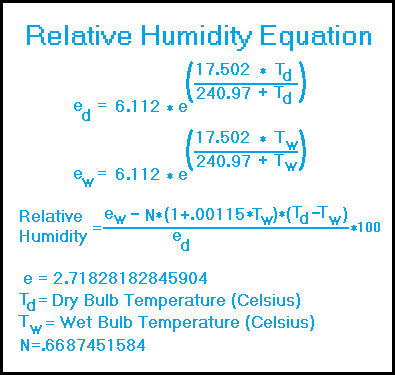Relative Humidity CalculatorRelative Humidity is measured using a device called a sling psychrometer. This consists of 2 side by side conventional thermometers, one of which (the wet bulb thermometer) has a wetted wick placed at the bottom, the other (the dry bulb thermometer) has no wetted wick.
When the psychrometer is swung around by its attached chain or handle, the air causes the water on the wick to evaporate which produces a lower temperature in the wet bulb thermometer. We now have a dry bulb and a wet bulb temperature which we can use to calculate relative humidity. The easiest way to do this is entering the dry and wet bulb temperatures into the calculator above and then click calculate. For example, if we have a dry bulb temperature of 20℃ and a wet bulb temperature of 15℃ what is the relative humidity? Using the calculator, we see that this is 58.4 %.

Now, let's try using the above equation. (The equation is so complex, for ease of use, it has been split into 3 parts.)

We have
dry bulb temp (Td) = 20℃ and wet bulb temp (Tw) = 15℃
What is the relative humidity?

Solving for ed
ed= 6.112 ✱ 2.71828(17.502 ✱ 20 ➗ 240.97 ✚ 20)

ed= 6.112 ✱ 2.71828(350.04 ➗ 260.97)

ed= 6.112 ✱ 2.71828(1.3413)

ed= 6.112 ✱ 3.8240

ed= 23.372

Solving for ew
ew= 6.112 ✱ 2.71828(17.502 ✱ 15 ➗ 240.97 ✚ 15)

ew= 6.112 ✱ 2.71828(262.53 ➗ 255.97)

ew= 6.112 ✱ 2.71828(1.0256)

ew= 6.112 ✱ 2.7888

ew= 17.045

Solving for relative humidity
rh = ([ew ➖ .66875 ✱ (1 ✚ .00115 ✱ Tw) ✱ (Td ➖ Tw)]➗ ed) ✱100

rh = ([17.045 ➖ .66875 ✱ (1 ✚ .00115 ✱ 15) ✱ (20 ➖ 15)] ➗ 23.372) ✱100

rh = [17.045 ➖ .66875 ✱ 1.01725 ✱ 5] ➗ .23372

rh = [17.045 ➖3.4014] ➗ .23372

rh = 58.376

rh = 58.4%

Significant Figures
The default numeric display is 3 significant figures.
For easier readability, numbers that are greater than .001 and less than 1000 are NOT in scientific notation.
You can change the display option by changing the number in the box above.
Entering a zero eliminates all formatting.

"R E T U R N"